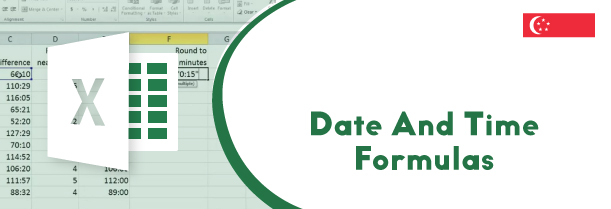# Lesson 15: Date and Time formulas in excel.

## How to use the Excel DATE And Time function in Excel!

This video is a part of free Excel Training Singapore, where I show you how to use Date and Time formulas in excel from the basics and also cover a lot of advanced Features.Except usually stores date and time as serial number and excel fetches these serial number to perform various functions such as HOUR, MINUTES, DAY, TODAY, WORKDAY, NETWORKDAY and many others to display the required output.

## Description of date and time formulas in excel.

Below mentioned are description of some of the most commonly used date and time formulas in excel along with their method of use.

• DAY – DAY function takes date serial number as input and gives the output as the day in the given month. This function takes only argument as its input which is the serial number.
• HOUR – HOUR function is used to return the hour of the given input. Hour function accepts only one argument as its input which is the serial number, it would recognize the number of hours that have elapsed that day and return that value.
• MINUTES – MINUTES function is used to return the number of minutes that have elapsed in the given day. This function takes only one argument as its input which is the serial number.
• DATE – DATE function in excel is used to return the serial number of the date when the user inputs the year, month and day value in the input section.
• TODAY – TODAY function returns the serial number of the current date. This function takes no argument as its input. This function keeps on getting updated on its own every time the worksheet is opened on a different date.

### How to use the DAY function?

DAY function takes serial number as input and return the output as the day in the given month. This function takes only one input. To see how this function works, follow the following mentioned steps –

• Start by typing the equals to sign followed by the function name which is DAY followed by the input which is serial number.
• In the given example, the serial number mentioned is 41699 and the output received on pressing ctrl with enter is 1, as this number represents 1st March 2014
• The serial number input can be copied as well from the previously prepared data table
• When the data inputted is in the from of a number, within double quotes, the data is treated as text only and the same output (1) is returned on pressing ctrl with enter

### How to use the DATE function?

DATE function in excel returns the serial number of the date when the user inputs the year, month and the day value in it.

• Start by typing the equals to symbol followed by the function name which is DATE. Inside the brackets type the inputs in the serial order year, month and day.
• Press ctrl along with enter to receive the output as the date’s serial number in the general format
• As excel stores the dates as serial numbers and the output can be changed to different formats just by changing the format to number, currency or any other
• The inputs can either be entered manually or the cell reference of the data previously mentioned can be selected as well.
• If the year is inputted in two digits and not in four digits then excel by default assumes it to be in the 19th century and returns the output accordingly

### How to use TODAY function?

TODAY function is used to return the serial number of the current date. This is a very volatile function and when applied to a worksheet having huge data, this function keeps on getting recalculated every time. To learn how this function works, follow the following mentioned steps –

• Start by typing the equals to symbol followed by the function name which is TODAY. As this function takes no argument as its input, just open and close the parenthesis and press enter. This function Will return the current date as the output
• If the user wishes to get a date ahead of the current date, simply type the number of days ahead in a separate table and select it’s reference after the parenthesis of the function have been closed. On pressing enter, the output so displayed will be ahead the current date.
• TODAY function can also be used to identify the day, month or year of the current day. To do this, just type the DAY function before TODAY function and press enter. The output so relieved will be the current date

#### Learn Microsoft Excel course in Singapore!

Microsoft excel holds great importance in almost all fields of work. To equip yourself with the finest knowledge from the experts just join a Best Excel Training Course in Singapore today!

In this video of this Free Excel Training Singapore, where you will learn about the following DATE and TIME formulas mentions below:

• DAY function
• HOUR function
• MINUTE function
• DATE function
• DATEVALUE function
• TODAY function
• NOW function
• WEEKDAY function
• NETWROKDAYS function
• INTL function
• WORKDAY function
• INTL function